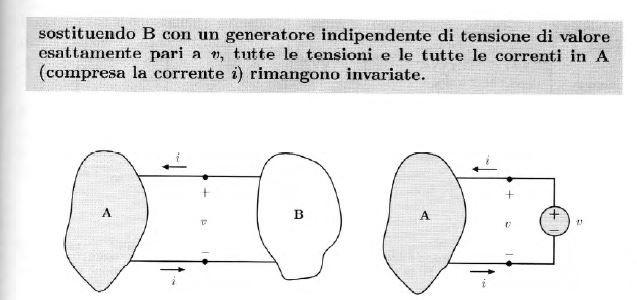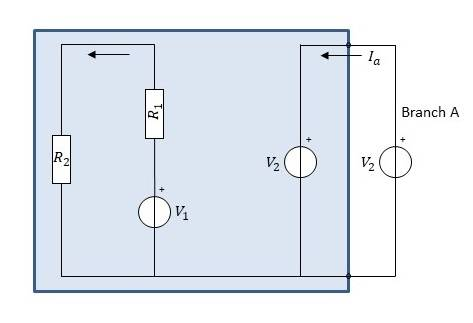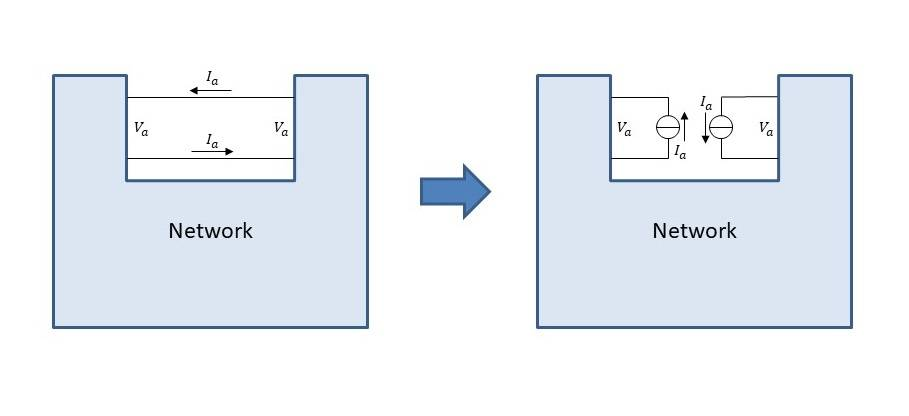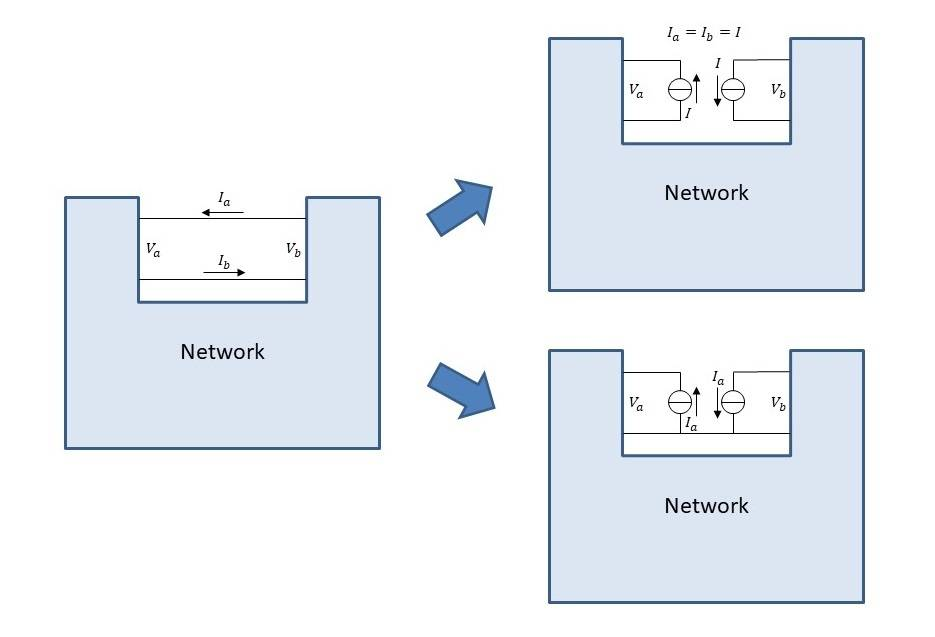# Circuit Theory - about the applicability of the substitution theorem

cianfa72
TL;DR Summary
About the conditions to be met for the applicability of substitution theorem in circuit theory
Hi,

I've a doubt about the applicability of the substitution theorem in circuit theory.

Consider the following picture (sorry for the Italian inside it)As far I can understand the substitution theorem can be applied to a given one-port element attached to a port (a port consists of two terminal that meets the condition: currents flowing into the two terminals are equal and opposite) when the following holds:
1. the starting network has at least one solution for the current/voltage of each graph link (one-port element)
2. the network obtained by replacing a given one-port with an independent generator having exactly the same port current (voltage) has an unique solution
Note that in above bullet 1, fixed a one-port element in the network, the starting network is required anyway to have a unique solution for a given value of that current (voltage) port. That means the starting network is allowed to have multiple solutions just for different values of that port current (voltage)

Does it make sense ?

Its been many years since learning the substitution theorem aka the compensation theorem. According to the substitution theorem if you have a network and in any branch, you know the current through it however determined you can replace all the impedances in that branch with a current source producing the current that you determined.

Likewise, if you know the voltage across an impedance in any branch however determined you may replace that impedance by a voltage source whose voltage is equal to the voltage that you determined.

cianfa72
This is my reasoning (based on the proof found in Basic Circuit theory Desoer & Kuh p.657)

Take a network and focus just on a specific branch (one-port element). Assume this initial network has multiple solutions in the sense that exist multiple values of that specific branch's current (or voltage) that are solutions of the network itself. Suppose then that for each of that given port current (or voltage) solution the values (solutions) of the other network branches currents/voltages are uniquely determined.

Consider now the network in which you replace that specific branch with a current source (or voltage source) producing that given value of current (or voltage). Now the current/voltage solutions of the initial network -- for that specific value (solution) of the port current (voltage)-- are actually solutions of the substituted network and -- in force of the uniqueness hypothesis about the substituted network -- we can conclude the (unique) solution of this final network is actually the solution of the network we started with.

Of course if you aim to extend this result to any chosen branch of the initial network (the network we started with) then the uniqueness of the initial network solution is actually required.

Last edited:
Hi,
I've a doubt about the applicability of the substitution theorem in circuit theory.
Do not first doubt the applicability of the substitution theorem. Take it as read.
First doubt your understanding of the applicability of the substitution theorem.

cianfa72
First doubt your understanding of the applicability of the substitution theorem.
Sure, that is indeed my general 'approach'...Take the following example.Here, even if the network has infinite solutions for the current through the branch A, we can successfully apply the theorem to the branch A: for each of the branch A current solution of the initial network, the replaced network has got an unique solution and that's of course the solution of the initial network for that specific branch A current value.

Again, if the theorem applies to any chosen branch of the network then the assumption of uniqueness of the initial network becomes required of course

Last edited:
cianfa72
Again, if the theorem applies to any chosen branch of the network then the assumption of uniqueness of the initial network becomes required of course

What do you think about, does it make sense ?

LvW
Yes - I think this is a precondition for the applicability of the theorem...

cianfa72
Yes - I think this is a precondition for the applicability of the theorem...
Ok. Take now the following scenarioAssume the overall network has a unique solution with equal currents ##I_a## through the drawn pair of wires: the definition of port is met -- even if in this case there exist no topological cut across them.

Let's assume the network drawn on the right has got an unique solution (there the pair of wires has been replaced by two current sources with value ##I_a## equal to the port current solution of the initial network).

Does it make sense to apply the substitution theorem also in this scenario ?

cianfa72
Does it make sense to apply the substitution theorem also in this scenario ?
Any comment on this ? Do you think it is actually the same case as before ?

cianfa72
Help !

Compared to my previous post #8 consider now the equivalence shown on the bottom right.I believe it applies to the general case where the currents ##I_a## and ##I_b## through the two wires are different (as before we assume the hypothesis of unique solution for the initial network and the replaced one).

Last edited: